# Jaeger, Francois

## Mapping planar graphs to odd cycles ★★★

Author(s): Jaeger

Conjecture   Every planar graph of girth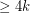has a homomorphism to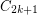.

Keywords: girth; homomorphism; planar graph

## The additive basis conjecture ★★★

Author(s): Jaeger; Linial; Payan; Tarsi

Conjecture   For every prime, there is a constant(possibly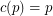) so that the union (as multisets) of anybases of the vector spacecontains an additive basis.

## A nowhere-zero point in a linear mapping ★★★

Author(s): Jaeger

Conjecture   Ifis a finite field with at least 4 elements andis an invertible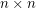matrix with entries in, then there are column vectorswhich have no coordinates equal to zero such that.

Keywords: invertible; nowhere-zero flow

## Petersen coloring conjecture ★★★

Author(s): Jaeger

Conjecture   Letbe a cubic graph with no bridge. Then there is a coloring of the edges ofusing the edges of the Petersen graph so that any three mutually adjacent edges ofmap to three mutually adjancent edges in the Petersen graph.

Keywords: cubic; edge-coloring; Petersen graph

## Jaeger's modular orientation conjecture ★★★

Author(s): Jaeger

Conjecture   Every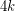-edge-connected graph can be oriented so that(mod) for every vertex.

Keywords: nowhere-zero flow; orientation